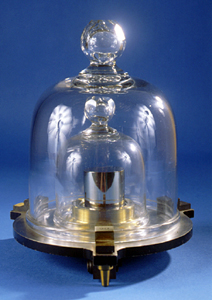# MassFigure 1. Replica of the national prototype kilogram standard no. K20 kept by the US government. It is 39 mm (1.5 inches) in diameter and 39 mm high.

Mass is a measure of how much matter is contained within an object. The SI unit for mass is the kilogram.

The mass of an object can be found by pushing on it with a known force F and dividing the size of that force by the resulting acceleration (a).

Mass is different from weight (force) in that its value does not change if gravity changes. For example, on Earth, where the acceleration due to gravity is 9.8 m/s2, a person who has 60 kg of mass weighs 588 Newtons (132 lbs). Whereas on the moon, where the acceleration due to gravity is about 1/6 of what it is on Earth, that same 60 kg person weighs just 98 Newtons (~22 lbs).